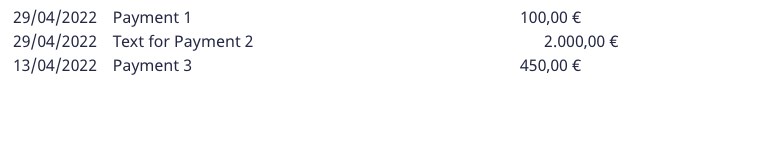0# State of payments

Hi,

i have a table (Payments) where I store all the payments that has been done for  each student.

Each payment has a date, a description, a payment method, an amount and an actual payer (mother, father, others...).

When I print the state of payments, I want to be able to see only the payments done by a selected payer for a selected student.

The 'join' function works fine for the purpose, but I get a badly aligned text which hurts the sight. I thought rPad and lPad could solve the problem, but it seems not...

This is the formula I used :

``````let xStud := record(Student,number(Person));
let xYear := record(SchoolYear,number(SchoolYear));
let xPayer := record(Payments,number(Payer));
switch Payer do
case 1:
join((select Payments)[Student = xStud and SchoolYear = xYear and Payer = xPayer].(Date + "    " + rpad (text(Description), 80, " ") + "    " + lpad(text(Amount), 10, " ")), "
")
case 2:
join((select Payments)[Student = xStud and SchoolYear = xYear and Payer = xPayer].(Date + "    " + rpad (text(Description), 80, " ") + "    " + lpad(text(Amount), 10, " ")), "
")
case 3:
join((select Payments)[Student = xStud and SchoolYear = xYear and Payer = xPayer].(Date + "    " + rpad (text(Description), 80, " ") + "    " + lpad(text(Amount), 10, " ")), "
")
case 4:
join((select Payments)[Student = xStud and SchoolYear = xYear].(Date + "    " + rpad (text(Description), 80, " ") + "    " + lpad(text(Amount), 10, " ")), "
")
default:
null
end
``````

and this is the alignment I get:Basically, I need to get stuff distanced and aligned correctly (eg: amount must be aligned on the right.